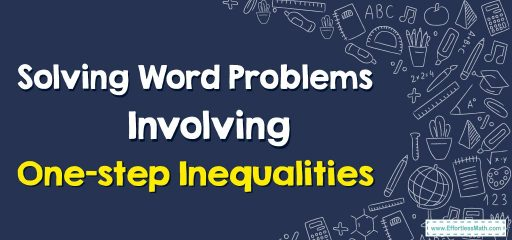# How to Solve Word Problems Involving One-step Inequalities?

In this step-by-step guide, you will learn how to solve word problems involving one-step inequalities.## A Step-by-step Guide to Solve Word Problems Involving One-step Inequalities

A word problem involving one-step inequalities is a type of mathematical problem that describes a situation using words and requires solving an inequality that can be solved with just one step.

In other words, the problem can be solved by performing one mathematical operation, such as adding, subtracting, multiplying, or dividing.

One-step inequalities involve comparing two values using one of the inequality symbols, such as $$<$$ (less than), $$>$$ (greater than), $$≤$$ (less than or equal to), or $$≥$$ (greater than or equal to).

The inequality symbol is used to represent the relationship between the two values in the problem.

Here’s a step-by-step guide to solving word problems involving one-step inequalities:

### Step 1: Understand the problem

Read the problem carefully and try to understand what it is asking you to do. Identify the unknown quantity and determine what it is that you are being asked to find.

Make note of any inequality symbols $$(>, <, ≥, ≤)$$ that are present in the problem.

### Step 2: Translate the problem into an inequality

Once you have understood the problem, translate it into an inequality. Use the inequality symbol that matches the problem’s direction.

For example, if the problem says “less than”, use $$<$$.

If it says “greater than”, use $$>$$.

### Step 3: Solve the inequality

Solve the inequality by performing the same operation on both sides of the inequality.

Remember that if you multiply or divide by a negative number, you must reverse the inequality symbol.

If you add or subtract a negative number, it is the same as adding or subtracting a positive number.

### Step 4: Check your solution

Check your solution by plugging it back into the original inequality.

If the inequality is true, your solution is correct. If not, recheck your work and try again.

### Step 5: Write the answer in a sentence

Write the solution to the problem in a complete sentence, using the correct units of measurement if applicable.

For example, let’s say you are given the problem: “Sam has at least $$20$$ in his pocket. Write an inequality to represent this situation.”

Step 1: Understand the problem

The unknown quantity is the amount of money Sam has in his pocket. We need to write an inequality to represent the situation.

Step 2: Translate the problem into an inequality

Sam has at least $$20$$ in his pocket, so the inequality can be written as: $$x\ge 20$$

Step 3: Solve the inequality

There is no need to solve inequality since it is already in its simplest form.

If we plug in any value greater than or equal to $$20$$, the inequality holds. For example, if Sam has $$25$$ in his pocket, the inequality $$25 ≥ 20$$ is true.

Step 5: Write the answer in a sentence

Sam has at least $$20$$ in his pocket.

### Solving Word Problems Involving One-step Inequalities – Examples 1

An athlete runs at least $$4$$ miles per day to train for a marathon. If the athlete has already run $$2$$ miles today, how many more miles does the athlete need to run to meet the daily training goal?

#### Solution:

To solve this problem, we can set up an inequality:

Miles run so far $$+$$ Additional miles run $$≥$$ Daily training goal

$$2 + x ≥ 4$$

We subtract $$2$$ from both sides to isolate the variable:

$$x ≥ 2$$

Therefore, the athlete needs to run at least $$2$$ more miles to meet the daily training goal.

### Solving Word Problems Involving One-step Inequalities – Examples 2

“Maria needs to save at least $$50$$ to buy a new pair of shoes. She has already saved $$30$$. How much more money does she need to save?”

#### Solution:

To solve this problem, we can set up an inequality:

Money saved so far $$+$$ Additional money saved $$≥$$ Total money needed

$$30 + x ≥ 50$$

We subtract $$30$$ from both sides to isolate the variable:

$$x ≥ 20$$

Therefore, Maria needs to save at least $$20$$ more to be able to buy the new pair of shoes.

### What people say about "How to Solve Word Problems Involving One-step Inequalities? - Effortless Math: We Help Students Learn to LOVE Mathematics"?

No one replied yet.

X
30% OFF

Limited time only!

Save Over 30%

SAVE $5 It was$16.99 now it is \$11.99